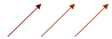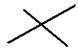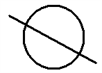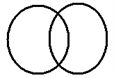# Combinations Questions

Combination: Combination is defined as the selection of r things that can be done out of total n things, combination is only selection, without arrangement.

Here the order is not important.

### Formula of Combination

The combination is denoted nCit is equal to n!/r!(n-r)!

Example: The Selection of a set of 11 players for a football team out of 20 players.

### Points to remember

1) Selection of ‘r’ things out of ‘n’ identical things can be done in only one way.

Selection of 4 blue balls from 10 blue balls can be done in only one way

(This is because whether you select the first four or last four or any four, you cannot differentiate between the balls)

2) Selection of ‘0’ or more things out of ‘n’ identical things can be done in ‘n+1’ ways.

Selection of ‘0’ or more balls out of 3 identical balls can be done in the following ways. (selecting no ball + 1 ball + 2 balls + 3 balls)

a) Selecting ‘0’ balls out of 3 identical balls (that is, not selecting anything).

This can be in just one way.

b) Selecting ‘1’ ball out of 3 identical balls.

This also can be done in just one way as balls are identical.

c) Selecting ‘2’ out of 3 balls. This also can be done in just one way as balls are identical.

d) Selecting all ‘3’ balls. This also can be done in just one way as balls are identical.

So total number of ways = 1+1+1+1= 4 ways So for ‘n’ identical things there are ‘n + 1’ ways of selecting zero or more things.

3) Selection of ‘1’ or more things out of ‘n’ identical things can be done in ‘n’ ways.

The cases will be all but (a) in the previous example totalling to 3 ways.

4) Selection of ‘r’ things out of ‘n’ distinct things can be done in n C r ways.

This is our basic formula for Combination.

5) Selection of ‘0’ or more things out of ‘n’ distinct things can be done in 2nways.

a) Selecting ‘0’ things from ‘n’ distinct things =>n C 0=1 way

b) Selecting‘1’ things from ‘n’ distinct things =>n C 1 ways

c) Selecting ‘2’ things from ‘n’ distinct things =>n C 2 ways

d) Selecting ‘3’ things from ‘n’ distinct things =>n C 3 ways

e) Selecting ‘n -1 ’ things from ‘n’ distinct things =>n C n -1 ways

f) Selecting ‘n ’ things from ‘n’ distinct things =>n C n ways

So adding all (‘0’ or more) ;

total number of ways = n C 0 + n C 1 + n C 2 + n C 3 + … + n C n-1 + n C n = 2n

6) Selection of ‘1’ or more things out of ‘n’ distinct things = 2n-1 ways. (Just remove the case (a) from the previous example)

Illustration:

Question 1) There are 5 Maths books, 4 Science books and 3 Literature books. How many collections can a person make taking at least one from each type

a) when all the books are different

b) when all the books are similar within a group

Solution:

a) When all the books are different, at least one of the 5 math books can be selected in (25-1 ways).

At least one of the 4 science books in (24-1) ways and selecting at least one of the 3 literature books in (23-1) ways.

b) When all the books are similar. The total number of ways of selection of one or more from 5 math books can be done in n=5 ways.

The number of ways of selecting one or more of the 4 science books is 4.

The number of ways in which one or more of the literature books can be selected is 3. Therefore the total number of ways is 5x4x3=60

Question 2) There are 5 maths books, 4 science books, 3 literature books. How many collections can a person make?

a) when all the books are different

b) when all the books are similar within a group

Solution:

(a) In this case when all the books are different, even 0 books can be selected.

Therefore the total number of ways in which the books can be selected in 212-1 =4095 (since 212=25 x24 x23 )

We remove 1 because we need to subtract that one case when no books are selected at all.

(b) Zero or more Maths books can be selected in 5+1 ways

Zero or more Science books can be selected in 4+1 ways

Zero or more Literature books can be selected in 3+1 ways

Total 6x5x4 = 120 ways,

but this includes a case where none of them is selected.

So subtract one. The required answer is 120-1=119

Prime Factorization of a Number-using Permutation & Combination

Now we will discuss an important feature of numbers using “Combination”.

If we have a number N such that N=am bncp where a, b, c are the prime factors then the number of factors of N = (m +1) (n +1) (p + 1)

Let us prove this using an example

Question 3) Find the number of factors of 2160?

Solution:

2160 can be represented in terms of its prime factors as 2160=243351 Thus the total number of factors will be a sum of the selection of ‘zero or more 2s’ (as 20=1, which is a factor), ‘zero or more 3s’ and ‘zero or more 5s’.

The 2’s can be selected in ?(4+1)=5 ways 3s?(3+1)=4 5s?(1+1)=2. Even if none are selected = 203050 =1.

1 is also a factor, that’s why we don’t subtract 1. Answer (4+1)(3+1)(1+1)=40

1.3.2 Applications of Combination Now we will discuss some questions that will help us to understand the application of Combination.

Illustration:

Question 4)

There are 10 points out of which 4 are collinear, how many straight lines can be formed?

Solution:

To form a line we need two points. The number of ways to select 2 points out of 10= 10C2.

But we have 4 collinear points and if we consider two points out of these four we can draw a line and if we consider another set of 2 points and join the same we will get the same straight line

So, all the 4C2 lines will be the same and will be counted as one. The required answer will be 10C2 – 4C2 + 1Number of Lines one line for

Lines with all due to 4 all 4C2 lines (the line which is formed of the four collinear points) 10 points points

Using similar logic, try solving the next question

Question 5) How many triangles can be formed from 10 points out of which 4 are collinear?

Solution:

To form a triangle, we need to select 3 non-collinear points.

So the required answer will be 10C3 (triangles formed using all 10 points) – 4C3 (triangles that were formed using the 4 collinear points, which is actually not possible)

Question 6) How many diagonals will be there for a polygon with ‘n’ sides?

Solution:

Diagonals are formed when we connect two points and get a line other than the side of the polygon.

So, we have ‘n’ points and we can connect 2 of ‘n’ points in nC2 ways.

This way we will get nC2 lines out of which ‘n’ will be the sides.

So the number of diagonals = nC2– n for a polygon with “n” sides.

Question 7) How many maximum points of intersection are possible from 8 lines and 4 circles.

Solution:

There will be three cases

i) Line and line intersectionThis can be done in 8C2 ways=28

ii) Line and circle intersectionOne line can be selected in 8 ways and one circle can be selected in 4 ways. The total number of ways = 8×4=32.

However, when a line and a circle intersect, we will get two points of intersection. Hence the total number of points of intersection are 32×2=64.

3) Circle and circleWhen two circles intersect, we get two points of intersection.

The 2 circles can be selected in 4C2 ways The points of intersection will be 4C2 x2 ways=12

Therefore the maximum number of points of intersection= 28+64+12=104